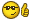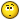# One = Two

@venkygec (783)
India
August 12, 2008 1:18pm CST
Let a = b a X a = b X b ---------------------------- squaring on both sides... a X a = a X b ---------------------------- since a=b (a X a) - (b X b) = (a X b) - (b X b) --- subtracting (b X b) on both sides... (a+b)(a-b) = b(a-b) ---------------------- since a2-b2=(a+b)-(a-b) and taking b common a + b = b -------------------------------- canceling a-b on both sides 2b = b ----------------------------------- since a=b 2 = 1 ------------------------------------ canceling b on both sides. So One = Two...Undestood!!!! How is it possible???Where is the mistake...1 person likes this
1 response
Yeah!!i can see that... Thats the reason im not getting any responses to this discusission... Still nobody could figure it out.... Anyways Thanks for the responseAnd keep posting on mylot....^_^